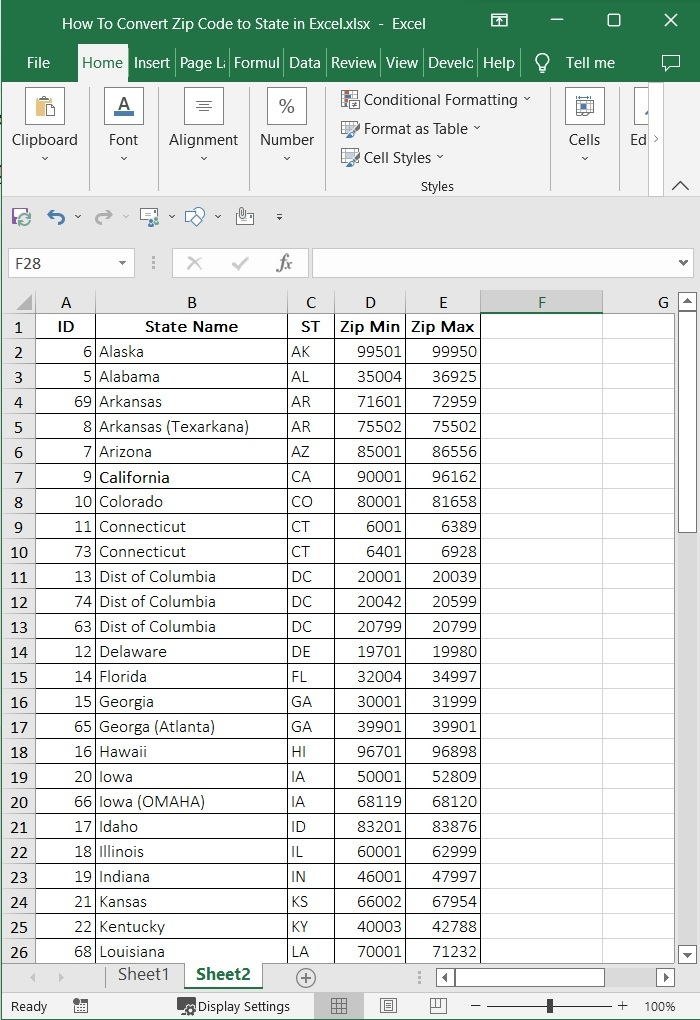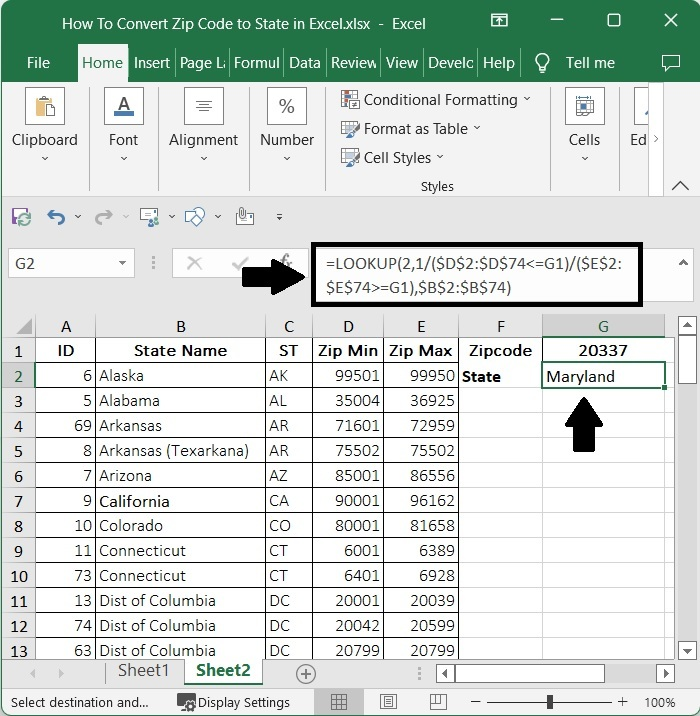# How to Convert Zip Code to State in Excel?

In Excel, you will occasionally be asked to convert a given zip code to the state name. A zip code can be used to uniquely identify a place based on a number. The zip code is mainly used for shipping the parcels. Every state has a starting and ending zip code according to its assigned order.

Read this tutorial to learn how you can convert a zip code to a state in Excel. Even though there is no direct formula available to complete the task, we can use the LOOKUP function to complete our task. We can use lookup when you need to find things in a table or a range by row. For example, look up the price of an automotive part by the part number or find an employee's name based on their employee ID. If we try to complete this manually, it can be a time-consuming process as we need to find every value in a long list of data.

## Converting Zip Code to State in Excel

Here we will use the VLOOKUP formula to get any one of the results, then use the auto-fill handle to get all the results. Let's look at a simple procedure for converting a zip code to a state name in Excel.

### Step 1

Let us consider a new excel sheet, then create a table that shows starting and ending zip codes with their state names, similar to the below image. We can find the list on the Internet.Then, in our case, click on an empty cell, H2, and enter the formula =LOOKUP(2,1/($D$2:$D$74=H1)/($E$2:$E$74>=H1), $B$2:$B$74) and press enter to get the result shown below.

In the formula, D2:D74, E2:E74, and B2:B74 are the ranges of the minimum zip code, the maximum zip code, and the state names, respectively, and H1 is the cell where the zip code is present on the sheet.

Empty cell > Formula > Enter## Conclusion

In this tutorial, we used a simple example to demonstrate how we can convert a zip code to a state name in Excel.

Updated on: 07-Mar-2023

2K+ Views